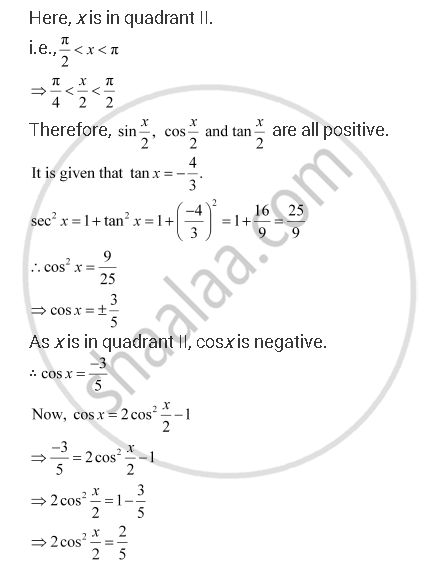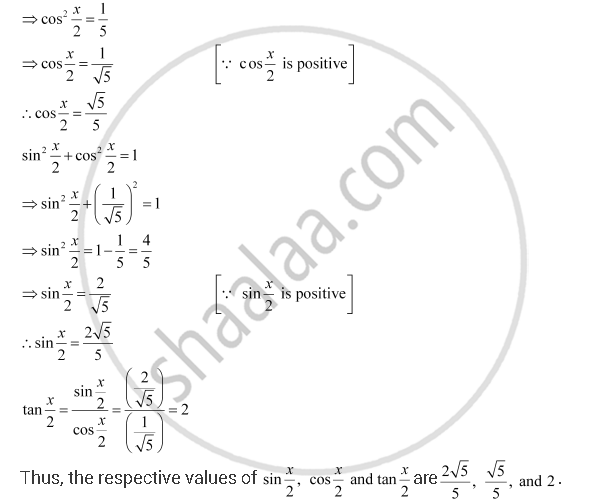CBSE (Arts) Class 11CBSE
Share

# Find Sin X/2, Cos X/2 and Tan X/2 of the Following Tan X = -4/3, X in Quadrant II - CBSE (Arts) Class 11 - Mathematics

ConceptDomain and Range of Trigonometric Functions

#### Question

Find sin x/2, cos x/2 and tan x/2 of the following

tan x =  -4/3, x in quadrant II

#### SolutionIs there an error in this question or solution?

#### APPEARS IN

NCERT Solution for Mathematics Textbook for Class 11 (2018 to Current)
Chapter 3: Trigonometric Functions
Q: 8 | Page no. 82

#### Video TutorialsVIEW ALL 

Solution Find Sin X/2, Cos X/2 and Tan X/2 of the Following Tan X = -4/3, X in Quadrant II Concept: Domain and Range of Trigonometric Functions.
S